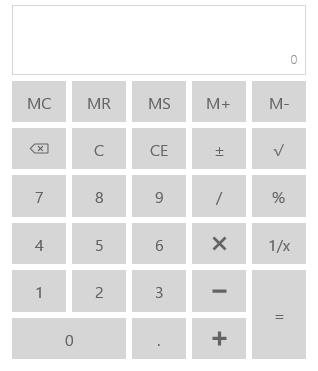# Getting Started with UWP Calculator (SfCalculator)

24 May 20211 minute to read

This section explains how to create a standalone calculator control that provide options to perform basic arithmetic operations.

Create a Universal Windows Platform project in Visual Studio and refer to the following assemblies.

• Syncfusion. SfInput.UWP

• Syncfusion.SfShared.UWP

1.Include the namespace for Syncfusion.SfInput.UWP assembly in MainPage.xaml

``````<Page xmlns="http://schemas.microsoft.com/winfx/2006/xaml/presentation"

xmlns:x="http://schemas.microsoft.com/winfx/2006/xaml"

xmlns:input="using:Syncfusion.UI.Xaml.Controls.Input">``````

2.Now add the `SfCalculator` control with a required optimal name using the included namespace

``<input:SfCalculator x:Name="calculator">``
``SfCalculator calculator = new SfCalculator();``
``Dim calculator As New SfCalculator()``

Above code example creates the calculator as follows and perform mathematical arithmetic operations using the provided buttons: